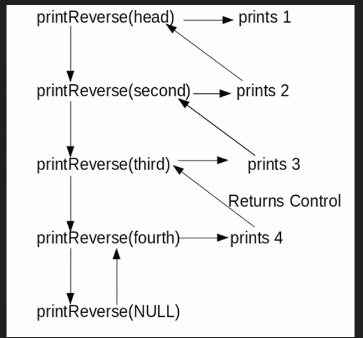Related Articles

# Print reverse of a Linked List without actually reversing

• Difficulty Level : Basic
• Last Updated : 12 May, 2021

Given a linked list, print reverse of it using a recursive function. For example, if the given linked list is 1->2->3->4, then output should be 4->3->2->1.
Note that the question is only about printing the reverse. To reverse the list itself see this
Difficulty Level: RookieAlgorithm

```printReverse(head)
1. call print reverse for head->next

Implementation:

## C++

 `// C++ program to print reverse of a linked list``#include ``using` `namespace` `std;` `/* Link list node */``class` `Node``{``    ``public``:``    ``int` `data;``    ``Node* next;``};` `/* Function to reverse the linked list */``void` `printReverse(Node* head)``{``    ``// Base case``    ``if` `(head == NULL)``    ``return``;` `    ``// print the list after head node``    ``printReverse(head->next);` `    ``// After everything else is printed, print head``    ``cout << head->data << ``" "``;``}` `/*UTILITY FUNCTIONS*/``/* Push a node to linked list. Note that this function``changes the head */``void` `push(Node** head_ref, ``char` `new_data)``{``    ``/* allocate node */``    ``Node* new_node = ``new` `Node();` `    ``/* put in the data */``    ``new_node->data = new_data;` `    ``/* link the old list off the new node */``    ``new_node->next = (*head_ref);` `    ``/* move the head to pochar to the new node */``    ``(*head_ref) = new_node;``}` `/* Driver code*/``int` `main()``{``    ``// Let us create linked list 1->2->3->4``    ``Node* head = NULL;``    ``push(&head, 4);``    ``push(&head, 3);``    ``push(&head, 2);``    ``push(&head, 1);``    ` `    ``printReverse(head);``    ``return` `0;``}` `// This code is contributed by rathbhupendra`

## C

 `// C program to print reverse of a linked list``#include``#include`` ` `/* Link list node */``struct` `Node``{``    ``int` `data;``    ``struct` `Node* next;``};`` ` `/* Function to reverse the linked list */``void` `printReverse(``struct` `Node* head)``{``    ``// Base case ``    ``if` `(head == NULL)``       ``return``;` `    ``// print the list after head node``    ``printReverse(head->next);` `    ``// After everything else is printed, print head``    ``printf``(``"%d  "``, head->data);``}`` ` `/*UTILITY FUNCTIONS*/``/* Push a node to linked list. Note that this function``  ``changes the head */``void` `push(``struct` `Node** head_ref, ``char` `new_data)``{``    ``/* allocate node */``    ``struct` `Node* new_node =``            ``(``struct` `Node*) ``malloc``(``sizeof``(``struct` `Node));`` ` `    ``/* put in the data  */``    ``new_node->data  = new_data;`` ` `    ``/* link the old list off the new node */``    ``new_node->next = (*head_ref);  `` ` `    ``/* move the head to pochar to the new node */``    ``(*head_ref)    = new_node;``}`` ` `/* Driver program to test above function*/``int` `main()``{``    ``// Let us create linked list 1->2->3->4``    ``struct` `Node* head = NULL;   ``    ``push(&head, 4);``    ``push(&head, 3);``    ``push(&head, 2);``    ``push(&head, 1);``  ` `    ``printReverse(head);``    ``return` `0;``}`

## Java

 `// Java program to print reverse of a linked list``class` `LinkedList``{``    ``Node head;  ``// head of list` `    ``/* Linked list Node*/``    ``class` `Node``    ``{``        ``int` `data;``        ``Node next;``        ``Node(``int` `d) {data = d; next = ``null``; }``    ``}` `    ``/* Function to print reverse of linked list */``    ``void` `printReverse(Node head)``    ``{``        ``if` `(head == ``null``) ``return``;` `        ``// print list of head node``        ``printReverse(head.next);` `        ``// After everything else is printed``        ``System.out.print(head.data+``" "``);``    ``}` `    ``/* Utility Functions */` `    ``/* Inserts a new Node at front of the list. */``    ``public` `void` `push(``int` `new_data)``    ``{``        ``/* 1 & 2: Allocate the Node &``                  ``Put in the data*/``        ``Node new_node = ``new` `Node(new_data);` `        ``/* 3. Make next of new Node as head */``        ``new_node.next = head;` `        ``/* 4. Move the head to point to new Node */``        ``head = new_node;``    ``}` `    ``/*Driver function to test the above methods*/``    ``public` `static` `void` `main(String args[])``    ``{``        ``// Let us create linked list 1->2->3->4``        ``LinkedList llist = ``new` `LinkedList();``        ``llist.push(``4``);``        ``llist.push(``3``);``        ``llist.push(``2``);``        ``llist.push(``1``);` `        ``llist.printReverse(llist.head);``    ``}``}``/* This code is contributed by Rajat Mishra */`

## Python3

 `# Python3 program to print reverse``# of a linked list` `# Node class``class` `Node:``    ` `    ``# Constructor to initialize``    ``# the node object``    ``def` `__init__(``self``, data):``        ` `        ``self``.data ``=` `data``        ``self``.``next` `=` `None``    ` `class` `LinkedList:``    ` `    ``# Function to initialize head``    ``def` `__init__(``self``):``        ``self``.head ``=` `None``    ` `    ``# Recursive function to print``    ``# linked list in reverse order``    ``def` `printrev(``self``, temp):``        ` `        ``if` `temp:``            ``self``.printrev(temp.``next``)``            ``print``(temp.data, end ``=` `' '``)``        ``else``:``            ``return``        ` `    ``# Function to insert a new node``    ``# at the beginning``    ``def` `push(``self``, new_data):``        ` `        ``new_node ``=` `Node(new_data)``        ``new_node.``next` `=` `self``.head``        ``self``.head ``=` `new_node` `# Driver code``llist ``=` `LinkedList()` `llist.push(``4``)``llist.push(``3``)``llist.push(``2``)``llist.push(``1``)` `llist.printrev(llist.head)``    ` `# This code is contributed by Vinay Kumar(vinaykumar71)`

## C#

 `// C# program to print reverse of a linked list``using` `System;` `public` `class` `LinkedList``{``    ``Node head; ``// head of list` `    ``/* Linked list Node*/``    ``class` `Node``    ``{``        ``public` `int` `data;``        ``public` `Node next;``        ``public` `Node(``int` `d)``        ``{``            ``data = d; next = ``null``;``        ``}``    ``}` `    ``/* Function to print reverse of linked list */``    ``void` `printReverse(Node head)``    ``{``        ``if` `(head == ``null``) ``return``;` `        ``// print list of head node``        ``printReverse(head.next);` `        ``// After everything else is printed``        ``Console.Write(head.data + ``" "``);``    ``}` `    ``/* Utility Functions */` `    ``/* Inserts a new Node at front of the list. */``    ``public` `void` `push(``int` `new_data)``    ``{``        ``/* 1 & 2: Allocate the Node &``                ``Put in the data*/``        ``Node new_node = ``new` `Node(new_data);` `        ``/* 3. Make next of new Node as head */``        ``new_node.next = head;` `        ``/* 4. Move the head to point to new Node */``        ``head = new_node;``    ``}` `    ``/*Driver function to test the above methods*/``    ``public` `static` `void` `Main(String []args)``    ``{``        ``// Let us create linked list 1->2->3->4``        ``LinkedList llist = ``new` `LinkedList();``        ``llist.push(4);``        ``llist.push(3);``        ``llist.push(2);``        ``llist.push(1);` `        ``llist.printReverse(llist.head);``    ``}``}` `// This code has been contributed by Rajput-Ji`

## Javascript

 ``

Output:

`4 3 2 1`

Time Complexity: O(n)